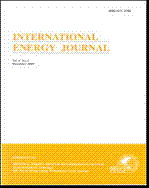## INTERNATIONAL ENERGY JOURNAL

Volume 19, No. 02, Month JUNE, Year 2019, Pages 77 - 88

Rudy Gianto

### Abstract

It is widely acknowledged that for modern electric distribution system embedded with wind turbine generators, the conventional load flow analysis needs to be reformulated to include the wind turbine generating system (WTGS) model in the analysis. This paper presents a simple method for modeling and incorporating fixed-speed WTGS into distribution system load flow analysis. The proposed model for the WTGS is developed based on the equivalent T-circuit of the asynchronous machine of the WTGS where only Kirchhoff and Ohm laws are used in forming the mathematical model. Unlike the previous model (i.e. PQ model), conversion from T-circuit to π-circuit is not necessary in the proposed model development. Moreover, since only one set of equations is needed to represent the WTGS, the proposed T-circuit model is therefore much simpler than the PQ model. The model is then integrated into the load flow algorithm to evaluate steady state operation of the distribution system and the WTGS. Test results on 33-node and 69-node distribution networks indicate that the proposed method is accurate and valid.

### Keywords

asynchronous generator, distribution system, induction generator, load flow analysis, wind turbine.

## INTERNATIONAL ENERGY JOURNAL

###### Published by : Asian Institute of TechnologyContributions welcome at : http://www.rericjournal.ait.ac.th